SYLLABUS  Previous: 3.3 Numerical quadrature  Up: 3 FINITE ELEMENT METHOD  Next: 3.5 Linear solvers

## 3.4 Implementation and solution

Slide : [ Scheme - Code - Run || VIDEO login]

Substituting the overlap integrals (3.3#eq.4) into (3.2#eq.5) yields the FEM scheme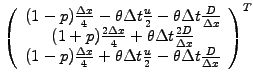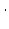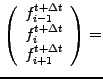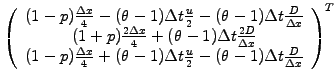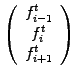(1)

Following an initialization where the initial condition is discretized with trivial projection on piecewise linear roof-top functions, the advection-diffusion scheme is implemented as
      BandMatrix a = new BandMatrix(3, f.length);
BandMatrix b = new BandMatrix(3, f.length);
double[]   c = new double[f.length];
double h   = dx;
double htm = h*(1-tune)/4;
double htp = h*(1+tune)/4;

for (int j=0; j<=n; j++) {
a.setL(j,   htm +h*(-0.5*beta -alpha)* theta    );
a.setD(j,2*(htp +h*(           alpha)* theta   ));
a.setR(j,   htm +h*( 0.5*beta -alpha)* theta    );
b.setL(j,   htm +h*(-0.5*beta -alpha)*(theta-1) );
b.setD(j,2*(htp +h*(           alpha)*(theta-1)));
b.setR(j,   htm +h*( 0.5*beta -alpha)*(theta-1) );
}
c=b.dot(f);                                 //Right hand side
c=c+b.getL(0)*f[n];                   // with periodicity
c[n]=c[n]+b.getR(n)*f;

fp=a.solve3(c);                             //Solve linear problem

Here again, the BandMatrix.solve3() method is used to compute a direct solution in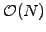operations, using an LU-factorization that will be examined with more details in the coming section. The entire scheme is illustrated below with the advection of a box function, calculated using a piecewise linear FEM discretization (with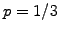) and a partly implicit time integration (with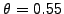).

 Numerical experiments: tunable finite-elements Vary the TimeImplicit parameter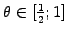and the TuneIntegr parameter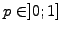to determine which combination has the smallest numerical damping. Repeat the study and try to minimize the phase errors. Introduce a finite physical Diffusion=1 and adjust the TimeImplicit and the TuneIntegr parameters to calculate a solution that is physically meaningful even with a large CFL number.

Having spent a considerable effort understanding the FEM formulation, isn't it frustrating to see how similar the code is to the implicit Crank-Nicholson FD scheme in sect.2.5? This should be the main argument for the community who still uses implicit finite difference schemes: with a little bit of thinking but the same computational cost, the finite element scheme is now considerably more flexibile: it is for example easy to densify the mesh16, modify the partly implicit time integration from centered to fully implicitand tune the integration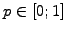. Convince yourself that Crank-Nicholson (2.5.1#eq.12) and this FEM scheme (3.4#eq.1) are indeed equivalent when using a homogeneous mesh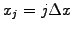, a time centered integration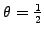and a trapezoidal quadrature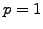.

SYLLABUS  Previous: 3.3 Numerical quadrature  Up: 3 FINITE ELEMENT METHOD  Next: 3.5 Linear solvers